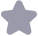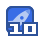### MATLAB数学建模与数学实验精品课件PPT分享-MATLAB精品教程课件 第8讲 最短路问题.rar收藏

MATLAB数学建模与数学实验精品课件PPT分享-MATLAB精品教程课件 第8讲 最短路问题.rar MATLAB数学建模与数学实验，精品课件PPT分享，共14讲，请从附件直接下载 MATLAB精品教程课件 第1讲 数学建模简介MATLAB精品教程课件 第2讲 MATLAB入门MATLAB精品教程课件 第3讲 MATLAB作图MATLAB精品教程课件 第4讲 线性规划MATLAB精品教程课件 第5讲 无约束优化MATLAB精品教程课件 第6讲 非线性规划MATLAB精品教程课件 第7讲 微分方程MATLAB精品教程课件 第8讲 最短路问题MATLAB精品教程课件 第9讲 行遍性问题MA

...展开详情
抢沙发
一个资源只可评论一次，评论内容不能少于5个字
weixin_39841365关注 私信 TA的资源
上传资源赚积分,得勋章
最新推荐